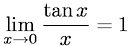Equations > Calculus > Limits > Limit of Tangent X over X as X Approaches Zero

### Limit of Tangent X over X as X Approaches ZeroLatex Code:

MathML Code:

 $\underset{x\to 0}{lim}\frac\mathrm{tan}xx=1$

MathType 5.0: